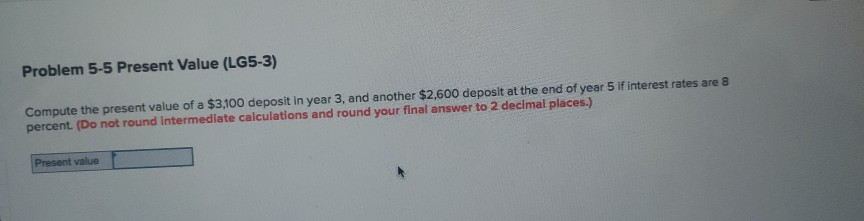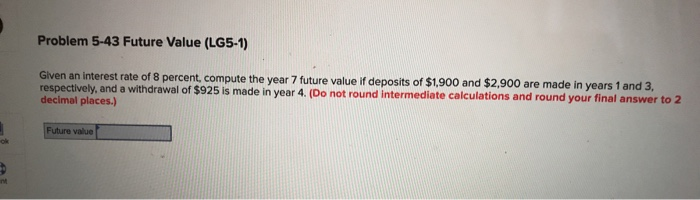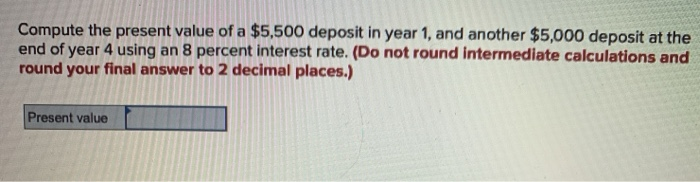Question

# Problem 5-1 Future Value (LG5-1) Compute the future value in year 9 of a \$3,300 deposit...

Problem 5-1 Future Value (LG5-1)

Compute the future value in year 9 of a \$3,300 deposit in year 1, and another \$2,800 deposit at the end of year 5 using a 9 percent interest rate. (Do not round intermediate calculations and round your final answer to 2 decimal places.)

Future value = _______

The future value is computed as shown below:

Future value = Present value ( 1 + r )n

= \$ 3,300 x 1.098 + \$ 2,800 x 1.094

= \$ 10,527.89 Approximately

#### Earn Coins

Coins can be redeemed for fabulous gifts.

Similar Homework Help Questions
• ### Problem 5-1 Future Value (LG5-1) Compute the future value in year 7 of a \$2,500 deposit...

Problem 5-1 Future Value (LG5-1) Compute the future value in year 7 of a \$2,500 deposit in year 1, and another \$2,000 deposit at the end of year 4 using a 8 percent interest rate. (Do not round intermediate calculations and round your final answer to 2 decimal places.)

• ### helpProblem 5-1 Future Value (LG5-1) 25 Compute the future value in year 7 of a...

Problem 5-1 Future Value (LG5-1) Compute the future value in year 7 of a \$2,200 deposit in year 1, and another \$1700 deposit at the end of year 4 using a 8 percent interest rate (Do not round intermediate calculations and round your final answer to 2 decimal places.) Problem 4 and 5-6 Present Value and Annuity Payments A local furniture store is advertising a deal in which you buy a \$4,200 living room set with three years before you need to make...

• ### Problem 5-5 Present Value (LG5-3) Compute the present value of a \$2,500 deposit in year 3,...

Problem 5-5 Present Value (LG5-3) Compute the present value of a \$2,500 deposit in year 3, and another \$2,000 deposit at the end of year 5 if interest rates are 8 percent. (Do not round intermediate calculations and round your final answer to 2 decimal places.)

• ### Problem 5-5 Present Value (LG5-3) Compute the present value of a \$3,100 deposit in year 3,...Problem 5-5 Present Value (LG5-3) Compute the present value of a \$3,100 deposit in year 3, and another \$2,600 deposit at the end of year 5 if interest rates are 8 percent (Do not round Intermediate calculations and round your final answer to 2 decimal places.) Present value

• ### Compute the future value in year 9 of a \$3,900 deposit in year 1, and another...

Compute the future value in year 9 of a \$3,900 deposit in year 1, and another \$3,400 deposit at the end of year 5 using a 9 percent interest rate. (Do not round intermediate calculations and round your final answer to 2 decimal places.) Future valueWhat is the future value of a \$990 annuity payment over five years if interest rates are 9 percent? (Do not round intermediate calculations and round your final answer to 2 decimal places.) Future value"What...

• ### Problem 5-43 Future Value (LG5-1) Given an interest rate of 8 percent, compute the year 7 future value if deposits...Problem 5-43 Future Value (LG5-1) Given an interest rate of 8 percent, compute the year 7 future value if deposits of \$1,900 and \$2,900 are made in years 1 and 3, respectively, and a withdrawal of \$925 is made in year 4. (Do not round Intermediate calculations and round your final answer to 2 decimal places.) Future value

• ### Compute the future value in year 8 of a \$5,000 deposit in year 1, and another...

Compute the future value in year 8 of a \$5,000 deposit in year 1, and another \$4,500 deposit at the end of year 3 using an 10 percent interest rate. (Do not round intermediate calculations and round your final answer to 2 decimal places.) Future value = _______

• ### Compute the future value in year 8 of a \$3,500 deposit in year 1 and another...

Compute the future value in year 8 of a \$3,500 deposit in year 1 and another \$3,000 deposit at the end of year 3 using a 10 percent interest rate. (Do not round intermediate calculations. Round your final answer to 2 decimal places.) Future value =

• ### Compute the future value in year 8 of a \$4,100 deposit in year 1 and another...

Compute the future value in year 8 of a \$4,100 deposit in year 1 and another \$3,600 deposit at the end of year 3 using an 10 percent interest rate. (Do not round intermediate calculations. Round your final answer to 2 decimal places.)   Future value \$

• ### Compute the present value of a \$5,500 deposit in year 1, and another \$5,000 deposit at...Compute the present value of a \$5,500 deposit in year 1, and another \$5,000 deposit at the end of year 4 using an 8 percent interest rate. (Do not round intermediate calculations and round your final answer to 2 decimal places.) Present value